# c++：-8

2022-05-20 14:21 2411 查看

##I/O流 （1）程序与外界环境的信息交换 当程序与外界环境进行信息交换时，存在着两个对象：程序中的对象、文件对象。 流是一种抽象，负责在数据的生产者和数据的消费者之间建立联系，并管理数据的流动。 （2）流对象与文件操作 程序建立一个流对象，指定这个流对象与某个文件对象建立连接程序操作流对象，流对象通过文件系统对所连接的文件对象产生作用。 （3）提取与插入 读操作在流数据抽象中被称为（从流中）提取，写操作被称为（向流中）插入。 （4）流类库结构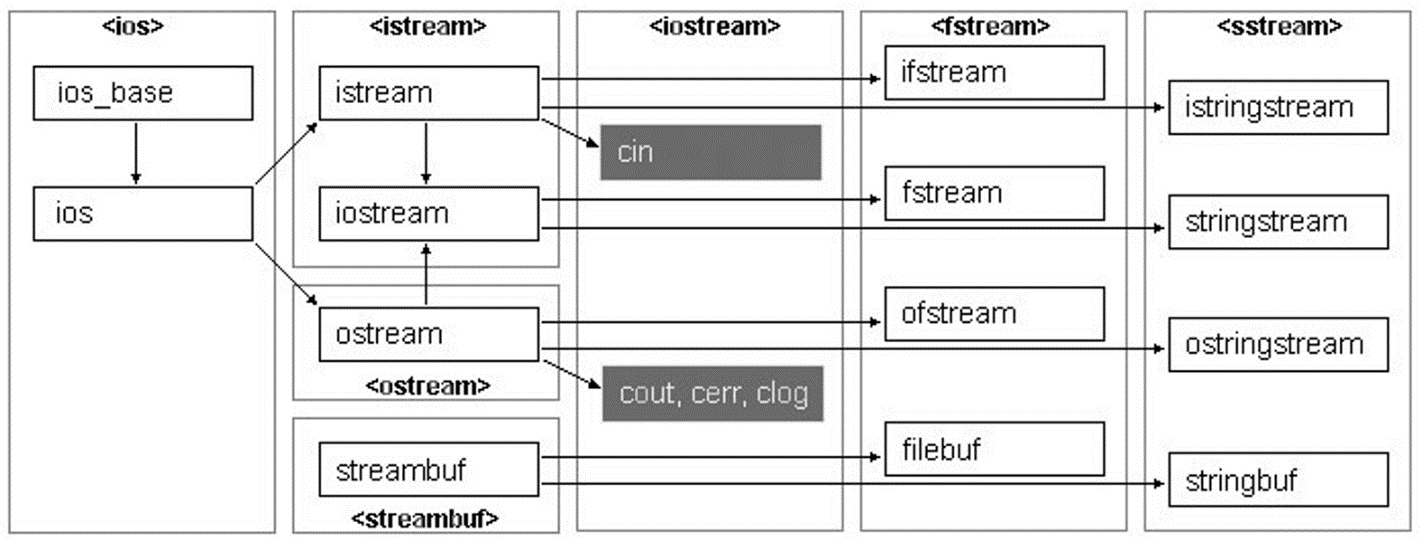（5）流类列表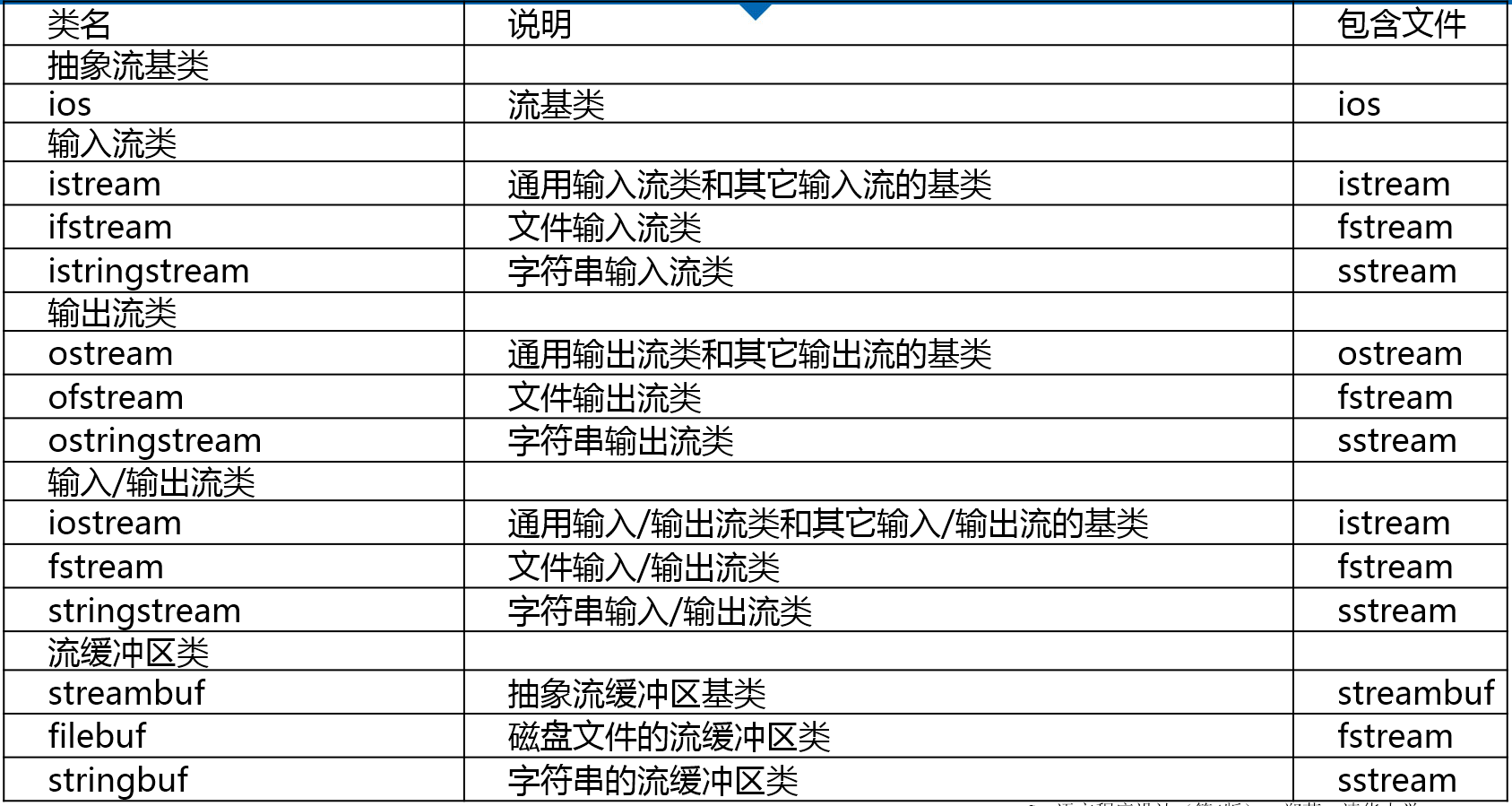##输出流 （1）三个重要的输出流：

• ostream
• ofstream
• ostringstream

（2）预先定义的输出流对象

• cout 标准输出
• cerr 标准错误输出，没有缓冲，发送给它的内容立即被输出。
• clog 类似于cerr，但是有缓冲，缓冲区满时被输出。

（3）标准输出换向

```ofstream fout("b.out");
streambuf*  pOld  =cout.rdbuf(fout.rdbuf());
//…
cout.rdbuf(pOld);```

（4）构造输出流对象

• ofstream类支持磁盘文件输出
• 如果在构造函数中指定一个文件名，当构造这个文件时该文件是自动打开的
`ofstream myFile("filename");`
• 可以在调用默认构造函数之后使用open成员函数打开文件
```ofstream myFile; //声明一个静态文件输出流对象
myFile.open("filename");   //打开文件，使流对象与文件建立联系```
• 在构造对象或用open打开文件时可以指定模式
`ofstream myFile("filename", ios_base::out | ios_base::binary);`

（5）文件输出流成员函数的三种类型

• 与操纵符等价的成员函数。
• 执行非格式化写操作的成员函数。
• 其它修改流状态且不同于操纵符或插入运算符的成员函数。

（6）文件输出流成员函数

• open函数 把流与一个特定的磁盘文件关联起来。 需要指定打开模式。
• put函数 把一个字符写到输出流中。
• write函数 把内存中的一块内容写到一个文件输出流中
• seekp和tellp函数 操作文件流的内部指针
• close函数 关闭与一个文件输出流关联的磁盘文件
• 错误处理函数 在写到一个流时进行错误处理 ###文本文件输出

（1）插入运算符

• 插入(<<)运算符
• 为所有标准C++数据类型预先设计的，用于传送字节到一个输出流对象。

（2）操纵符（manipulator）

• 插入运算符与操纵符一起工作 控制输出格式。
• 很多操纵符都定义在ios_base类中（如hex()）、头文件（如setprecision()）
• 控制输出宽度 在流中放入setw操纵符或调用width成员函数为每个项指定输出宽度。
• setw和width仅影响紧随其后的输出项，但其它流格式操纵符保持有效直到发生改变。
• dec、oct和hex操纵符设置输入和输出的默认进制。

（3）举例

• 使用width控制输出宽度
```#include <iostream>
using namespace std;

int main() {
double values[] = { 1.23, 35.36, 653.7, 4358.24 };
for(int i = 0; i < 4; i++) {
cout.width(10);//控制输出宽度，默认右对齐
cout << values[i] << endl;
}
return 0;
}

1.23
35.36
653.7
4358.24```
• 使用setw操纵符指定宽度
```#include <iostream>
#include <iomanip>
#include <string>
using namespace std;

int main() {
double values[] = { 1.23, 35.36, 653.7, 4358.24 };
string names[] = { "Zoot", "Jimmy", "Al", "Stan" };
for (int i = 0; i < 4; i++)
cout << setw(6) << names[i]  //和width的功能是一样的，默认右对齐
<< setw(10) << values[i] << endl;
return 0;
}

Zoot      1.23
Jimmy     35.36
Al     653.7
Stan   4358.24```
• 设置对齐方式
```#include <iostream>
#include <iomanip>
#include <string>
using namespace std;

int main() {
double values[] = { 1.23, 35.36, 653.7, 4358.24 };
string names[] = { "Zoot", "Jimmy", "Al", "Stan" };
for (int i=0;i<4;i++)
cout << setiosflags(ios_base::left)//设置左对齐
<< setw(6) << names[i]
<< resetiosflags(ios_base::left)//取消对齐方式，默认右对齐
<< setw(10) << values[i] << endl;
return 0;
}

Zoot        1.23
Jimmy      35.36
Al         653.7
Stan     4358.24```

（4）setiosflags操纵符

• 这个程序中，通过使用带参数的setiosflags操纵符来设置左对齐，setiosflags定义在头文件iomanip中。
• 参数iosbaseleft是iosbase的静态常量，因此引用时必须包括**ios_base**前缀。
• 这里需要用resetiosflags操纵符关闭左对齐标志。setiosflags不同于width和setw，它的影响是持久的，直到用resetiosflags重新恢复默认值时为止 。
• setiosflags的参数是该流的格式标志值，可用按位或（|）运算符进行组合

（5）setiosflags的参数（流的格式标）

• ios_base::skipws 在输入中跳过空白 。
• ios_base::left 左对齐值，用填充字符填充右边。
• ios_base::right 右对齐值，用填充字符填充左边（默认对齐方式）。
• ios_base::internal 在规定的宽度内，指定前缀符号之后，数值之前，插入指定的填充字符。
• ios_base::dec 以十进制形式格式化数值（默认进制）。
• ios_base::oct 以八进制形式格式化数值 。
• ios_base::hex 以十六进制形式格式化数值。
• ios_base::showbase 插入前缀符号以表明整数的数制。
• ios_base::showpoint 对浮点数值显示小数点和尾部的0 。
• ios_base::uppercase 对于十六进制数值显示大写字母A到F，对于科学格式显示大写字母E 。
• ios_base::showpos 对于非负数显示正号（“+”）。
• ios_base::scientific 以科学格式显示浮点数值。
• ios_base::fixed 以定点格式显示浮点数值（没有指数部分） 。
• ios_base::unitbuf 在每次插入之后转储并清除缓冲区内容。

（6）精度

• 浮点数输出精度的默认值是6，例如：3466.98。
• 要改变精度：setprecision操纵符（定义在头文件iomanip中）。
• 如果不指定fixed或scientific，精度值表示有效数字位数
• 如果设置了iosbasefixed或iosbasescientific精度值表示小数点之后的位数

（7）举例

• 控制输出精度——未指定fixed或scientific
```#include <iostream>
#include <iomanip>
#include <string>
using namespace std;

int main() {
double values[] = { 1.23, 35.36, 653.7, 4358.24 };
string names[] = { "Zoot", "Jimmy", "Al", "Stan" };
for (int i=0;i<4;i++)
cout << setiosflags(ios_base::left)//设置左对齐
<< setw(6) << names[i]
<< resetiosflags(ios_base::left)//清除左对齐设置
<< setw(10) << setprecision(1) << values[i] << endl; //没有设置fixed或scientific，则精度表示有效位数
return 0;
}

Zoot           1
Jimmy      4e+01
Al         7e+02
Stan       4e+03```
• 控制输出精度——指定fixed
```#include <iostream>
#include <iomanip>
#include <string>
using namespace std;
int main() {
double values[] = { 1.23, 35.36, 653.7, 4358.24 };
string names[] = { "Zoot", "Jimmy", "Al", "Stan" };
cout << setiosflags(ios_base::fixed); //设置以fixed形式输出，则精度为小数点后的位数
for (int i=0;i<4;i++)
cout << setiosflags(ios_base::left)//左对齐设置
<< setw(6) << names[i]
<< resetiosflags(ios_base::left)//清除左对齐设置
<< setw(10) << setprecision(1) << values[i] << endl;
return 0;
}

Zoot         1.2
Jimmy       35.4
Al         653.7
Stan      4358.2```
• 控制输出精度——指定scientific
```#include <iostream>
#include <iomanip>
#include <string>
using namespace std;
int main() {
double values[] = { 1.23, 35.36, 653.7, 4358.24 };
string names[] = { "Zoot", "Jimmy", "Al", "Stan" };
cout << setiosflags(ios_base::scientific);//设置以scientific形式输出，则精度为小数点后的位数
for (int i=0;i<4;i++)
cout << setiosflags(ios_base::left)//左对齐设置
<< setw(6) << names[i]
<< resetiosflags(ios_base::left)//清除左对齐设置
<< setw(10) << setprecision(1) << values[i] << endl;
return 0;
}

Zoot     1.2e+00
Jimmy    3.5e+01
Al       6.5e+02
Stan     4.4e+03```

###二进制文件输出 （1）二进制文件流（ofstream）

• 使用ofstream构造函数中的模式参量指定二进制输出模式；
• 以通常方式构造一个流，然后使用setmode成员函数，在文件打开后改变模式；
• 通过二进制文件输出流对象完成输出。

（2）举例：向二进制文件输出

```#include <fstream>
using namespace std;
struct Date {
int mon, day, year;
};
int main() {
Date dt = { 6, 10, 92 };
ofstream file("date.dat", ios_base::binary);//打开二进制文件
file.write(reinterpret_cast<char *>(&dt),sizeof(dt));//写入数据
file.close();
return 0;
}```

###字符串输出

（1）字符串输出流（ ostringstream

• 用于构造字符串
• 功能 支持ofstream类的除open、close外的所有操作 str函数可以返回当前已构造的字符串
• 典型应用 将数值转换为字符串

（2）举例：用ostringstream将数值转换为字符串

```#include <iostream>
#include <sstream>
#include <string>
using namespace std;

//函数模板toString可以将各种支持“<<“插入符的类型的对象转换为字符串。
template <class T>
inline string toString(const T &v) {
ostringstream os;   //创建字符串输出流
os << v;        //将变量v的值写入字符串流
return os.str();    //返回输出流生成的字符串
}

int main() {
string str1 = toString(5);
cout << str1 << endl;
string str2 = toString(1.2);
cout << str2 << endl;
return 0;
}

5
1.2```

##输入流 （1）重要的输入流类

• istream类最适合用于顺序文本模式输入。cin是其实例。
• ifstream类支持磁盘文件输入。
• istringstream：支持字符串输入

（2）构造输入流对象

• 如果在构造函数中指定一个文件名，在构造该对象时该文件便自动打开。
`ifstream myFile("filename");`
• 在调用默认构造函数之后使用open函数来打开文件。
```ifstream myFile; //建立一个文件流对象
myFile.open("filename"); //打开文件"filename”```
• 打开文件时可以指定模式
`ifstream myFile("filename", iosbase::in | iosbase::binary);`

（3）使用提取运算符从文本文件输入

• 提取运算符(>>)对于所有标准C++数据类型都是预先设计好的。
• 是从一个输入流对象获取字节最容易的方法。
• ios类中的很多操纵符都可以应用于输入流。但是只有少数几个对输入流对象具有实际影响，其中最重要的是进制操纵符dec、oct和hex。

（4）输入流相关函数

• open 把该流与一个特定磁盘文件相关联。
• get 功能与提取运算符（>>）很相像，主要的不同点是get函数在读入数据时包括空白字符。
• getline 功能是从输入流中读取多个字符，并且允许指定输入终止字符，读取完成后，从读取的内容中删除终止字符。
• seekg 用来设置文件输入流中读取数据位置的指针。
• tellg 返回当前文件读指针的位置。
• close 关闭与一个文件输入流关联的磁盘文件。

###举例 （1）get函数应用举例

```#include <iostream>
using namespace std;
int main() {
char ch;
while ((ch = cin.get()) != EOF)//键盘输入一个字符
cout.put(ch);//输出
return 0;
}```

（2）为输入流指定一个终止字符

```#include <iostream>
#include <string>
using namespace std;
int main() {
string line;
cout << "Type a line terminated by 't' " << endl;
getline(cin, line, 't');//输入一行字符，以t字符截止
cout << line << endl;
return 0;
}```

（3）从文件读一个二进制记录到一个结构中

```#include <iostream>
#include <fstream>
#include <cstring>
using namespace std;

struct SalaryInfo {
unsigned id;
double salary;
};
int main() {
SalaryInfo employee1 = { 600001, 8000 };
ofstream os("payroll", ios_base::out | ios_base::binary);
os.write(reinterpret_cast<char *>(&employee1), sizeof(employee1));//写入
os.close();

ifstream is("payroll", ios_base::in | ios_base::binary);
if (is) {
SalaryInfo employee2;
cout << employee2.id << " " << employee2.salary << endl;
} else {
cout << "ERROR: Cannot open file 'payroll'." << endl;
}
is.close();
return 0;
}```

（4）用seekg函数设置位置指针

```#include <iostream>
#include <fstream>
using namespace std;

int main() {
int values[] = { 3, 7, 0, 5, 4 };
ofstream os("integers", ios_base::out | ios_base::binary);
os.write(reinterpret_cast<char *>(values), sizeof(values));//写入
os.close();

ifstream is("integers", ios_base::in | ios_base::binary);
if (is) {
is.seekg(3 * sizeof(int));//跳到第四个数
int v;
cout << "The 4th integer in the file 'integers' is " << v << endl;
} else {
cout << "ERROR: Cannot open file 'integers'." << endl;
}
return 0;
}

（5）读一个文件并显示出其中0元素的位置

```#include <iostream>
#include <fstream>
using namespace std;

int main() {
ifstream file("integers", ios_base::in | ios_base::binary);
if (file) {
while (file) {//读到文件尾file为0
streampos here = file.tellg();//here记录位置，streampos可以看作int类型
int v;
if (file && v == 0)
cout << "Position " << here << " is 0" << endl;
}
} else {
cout << "ERROR: Cannot open file 'integers'." << endl;
}
file.close();
return 0;
}

Position 8 is 0```

###字符串输入

（1）字符串输入流（ istringstream）

• 用于从字符串读取数据
• 在构造函数中设置要读取的字符串

（2）功能

• 支持ifstream类的除open、close外的所有操作

（3）典型应用

• 将字符串转换为数值

（4）举例：用istringstream将字符串转换为数值

```#include <iostream>
#include <string>
#include <sstream>

using namespace std;

template <class T>
inline T fromString(const string &str) {
istringstream is(str);  //创建字符串输入流
T v;
is >> v;    //从字符串输入流中读取变量v
return v;   //返回变量v
}

int main() {
int v1 = fromString<int>("5");
cout << v1 << endl;
double v2 = fromString<double>("1.2");
cout << v2 << endl;
return 0;
}

5
1.2```

##输入/输出流 （1）两个重要的输入/输出流

• 一个iostream对象可以是数据的源或目的。
• 两个重要的I/O流类都是从iostream派生的，它们是fstream和stringstream。这些类继承了前面描述的istream和ostream类的功能。

（2）fstream类

• fstream类支持磁盘文件输入和输出
• 如果需要在同一个程序中从一个特定磁盘文件读并写到该磁盘文件，可以构造一个fstream对象。
• 一个fstream对象是有两个逻辑子流的单个流，两个子流一个用于输入，另一个用于输出。

（3）stringstream类

• stringstream类支持面向字符串的输入和输出
• 可以用于对同一个字符串的内容交替读写，同样是由两个逻辑子流构成。

##程序 ###宏定义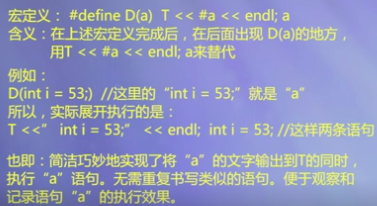###向程序传递参数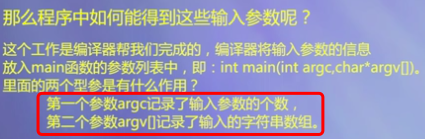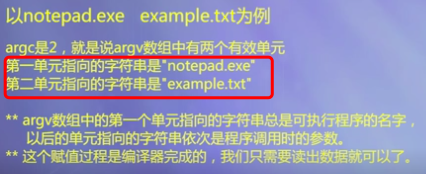（1）输入输出格式

```#include <fstream>
using namespace std;
#define D(a) T << #a << endl; a  //将a中的值写入文件
ofstream T("output.out"); //打开文件

int main() {
D(int i = 53;)
D(float f = 4700113.141593;)
char* s = "Is there any more?";

D(T.setf(ios::unitbuf);)

D(T.setf(ios::showbase);)
D(T.setf(ios::uppercase);)
D(T.setf(ios::showpos);)
D(T << i << endl;)
D(T.setf(ios::hex, ios::basefield);)
D(T << i << endl;)
D(T.unsetf(ios::uppercase);)
D(T.setf(ios::oct, ios::basefield);)
D(T << i << endl;)
D(T.unsetf(ios::showbase);)
D(T.setf(ios::dec, ios::basefield);)
D(T.fill('0');)
D(T << "fill char: " << T.fill() << endl;)
D(T.width(8);)
T << i << endl;
D(T.width(8);)
T << i << endl;
D(T.width(8);)
T << i << endl;
D(T << i << endl;) // Without width(10)

D(T.unsetf(ios::showpos);)
D(T.setf(ios::showpoint);)
D(T << "prec = " << T.precision() << endl;)
D(T.setf(ios::scientific, ios::floatfield);)
D(T << endl << f << endl;)
D(T.setf(ios::fixed, ios::floatfield);)
D(T << f << endl;)
D(T.setf(0, ios::floatfield);)
D(T << f << endl;)
D(T.precision(16);)
D(T << "prec = " << T.precision() << endl;)
D(T << endl << f << endl;)
D(T.setf(ios::scientific, ios::floatfield);)
D(T << endl << f << endl;)
D(T.setf(ios::fixed, ios::floatfield);)
D(T << f << endl;)
D(T.setf(0, ios::floatfield);)
D(T << f << endl;)

D(T.width(8);)
T << s << endl;
D(T.width(36);)
T << s << endl;
D(T.width(36);)
T << s << endl;

D(T.unsetf(ios::showpoint);)
D(T.unsetf(ios::unitbuf);)
}```

（2）

```#include <iostream>
#include <fstream>
using namespace std;

int main(int argc, char* argv[])
{
ifstream in;
in.open(argv, ios::binary);//打开argv内的数组元素
if (!in) {
cout << "Cannot open file.";
return 1;
}
const int bsz = 1024;
char buf[bsz];
int line = 0;
while(in.getline(buf, bsz)) {
cout << ++line << ": " << buf << endl;
}
return 0;
}```

##习题 （1）定义一个结构体

```struct Date{
int mon,day,year;
};```

```istringstream istr("5 1.2");
int a;
float b;
istr >> a >> b;```